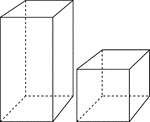2 Rectangular Prisms

Illustration of 2 right rectangular prisms. The bases are congruent, but the height of the smaller prism…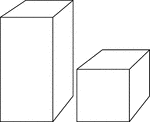2 Rectangular Prisms

Illustration of 2 right rectangular prisms. The bases are congruent, but the height of the smaller prism…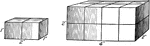Similar Rectangular Solids

Illustration of a 1 in. by 2 in. by 1 in. rectangular solid that is similar to a 2 in. by 4 in. by 2…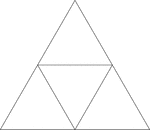Equilateral Triangle Inscribed In An Equilateral Triangle

Illustration of an equilateral triangle inscribed in an equilateral triangle. The smaller triangle is…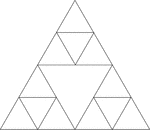Equilateral Triangles Inscribed In An Equilateral Triangle

Illustration of an equilateral triangle inscribed in an equilateral triangle by joining the midpoints…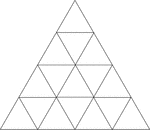Equilateral Triangles Inscribed In Equilateral Triangles

Illustration of an equilateral triangle inscribed in an equilateral triangle by joining the midpoints…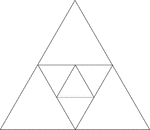Equilateral Triangles Inscribed In Equilateral Triangles

Illustration of an equilateral triangle inscribed in an equilateral triangle by joining the midpoints…